# What is Similarity?

Go back to  'Similar-Triangles'

In an earlier chapter, we have studied the concept of congruence. Two geometrical figures are said to be congruent when they can be superimposed on each other exactly. In other words, two congruent figures are alike in every respect.

In this chapter, we will study the concept of similarity. The simplest description of similarity is: two figures are similar if they have the same shape (they may not have the same size). For example, two circles (of any radii) will always be similar:If the two circles happen to have equal radii, they will be congruent as well. Two squares (of any side lengths) will always be similar: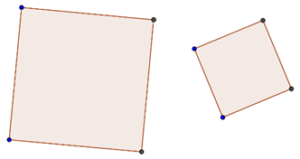They will be congruent only then their side lengths are equal to each other, but they will always be similar.

Suppose that you have a picture or figure of an arbitrary quadrilateral. You shrink that picture, and compare it with the original quadrilateral: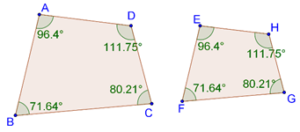What do you observe? The two figures have exactly the same shape, but not the same size. These two quadrilaterals are similar, but not congruent. Note how all the angles are preserved.

You can intuitively think of similarity as follows: two figures A and B will be similar if B can be enlarged or shrunk (by a certain factor) to make it congruent with A. If A and B are congruent to begin with, then they are obviously similar as well. Thus, similarity is more general than congruent, that is, congruence is a special case of similarity.

Let’s consider some more examples of similar figures.

Example 1: Two similar triangles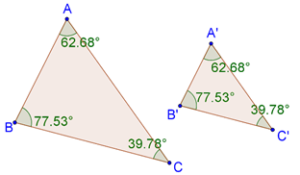Note how the (corresponding) angles of the two triangles are exactly the same. Can you figure out what the relation is between their corresponding sides?

Example 2: Two similar pentagons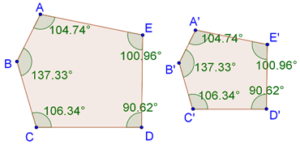Once again, observe how the corresponding angles are equal.

Example 3: Two similar arcs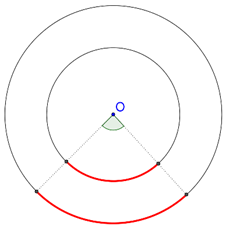The two arcs under consideration have been highlighted. The two arcs are similar because they subtend the same angle at the centre O of their parent circles.

It should be clear to you by now that two figures are similar if one can be obtained from the other by uniform scaling, that is, by uniform enlarging or shrinking.

Learn math from the experts and clarify doubts instantly

• Instant doubt clearing (live one on one)
• Learn from India’s best math teachers
• Completely personalized curriculum